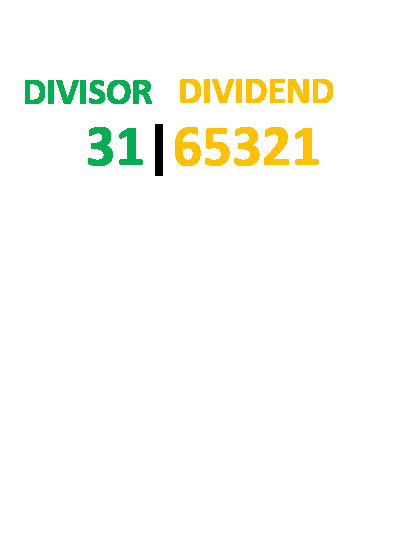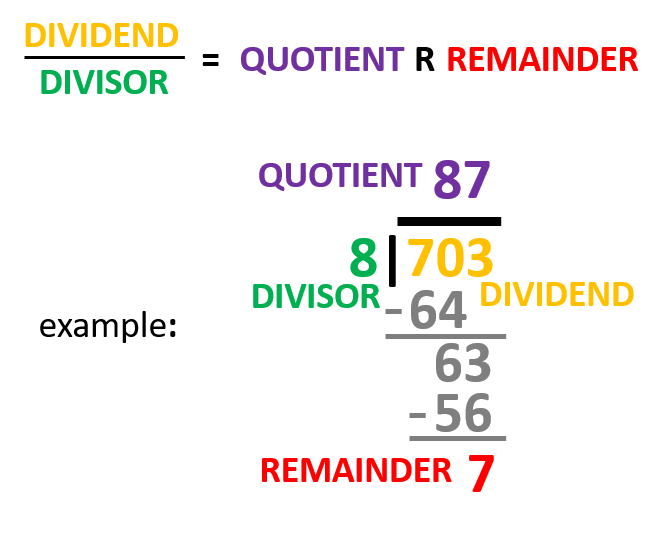# Long Division Calculator

Created by Wojciech Sas, PhD
Reviewed by Bogna Szyk and Jack Bowater
Last updated: Feb 02, 2023

Welcome to the long division calculator, the tool that helps you understand how to do long division with decimals. Read on to learn how to solve long division problems and how to deal with long division with remainders. You can also find a long division example, complete with thoroughly explained long division steps.

If you need just a quick answer to your problem, you may find Omni's remainder calculator a perfect choice.

## Long division with remainders

As we want to learn how to do long division, let's start with the basics - definitions.

Typically, we want to find a result, which is the ratio of two numbers. These two numbers are a dividend (not to be confused with dividend in finance) and a divisor. We can also write it as:

result = dividend / divisor.

We can write the result in various forms: as a fraction, a decimal (converted from the fraction), or as a combination of two numbers, a quotient, and a remainder. The latter is the essence of long division problems.

If you want to leave your result in the fractional form, you might find our ratio calculator quite helpful: it simplifies ratios quickly and easily!

As a special case, if you want to determine the divisibility of a positive integer by 3 or 9, you can directly use the digit sum property to figure it out!

## How to do long division with decimals?

The whole process is relatively simple as you have to repeat the long division steps:

1. As we are going to divide each part of the dividend on its own, we need to break it up. Begin by looking at how many digits your divisor has. This is how many digits we take from the left-hand side of the dividend to start working with. For example, if we divide 378 by 14, we will look at the 37 part of 378. We will call the numbers we take n₁.

2. If n₁ is smaller than the divisor, take the next digit from the dividend too.

3. Divide this value by the divisor and round the result down to the nearest whole number. This is the first digit of the quotient.

4. Multiply that digit by the divisor. Let's call this n₂.

5. Subtract n₁ and n₂. We usually receive some remainder.

6. With this new number, write the next digit to the right from the dividend (step 1) to the right of this value, which is our new n₁.

7. Continue these long division steps until you run out of digits in the dividend.

8. When you use the last digit from the dividend, and the difference n₁ - n₂ yields a non-zero value, that's the final remainder

9. You may continue by writing down further trailing zeros to obtain greater precision and to have more significant figures. But be careful; sometimes, it never ends, like for recurring decimals!

By the way, if you are interested in getting only the remainder, you can use the modulo claculator because the equality dividend mod divisor = remainder is always true.

## Long division example with steps

As we've already learned the theory, let's try to solve a particular long division example and see how our long division calculator works. In this case, let's see how to do a long division of 65321 and 31:• Take the first two digits from the dividend, 65. Divide this value by 31, and round it down to the whole number, which gives us 2. Write it above as the first digit of the quotient.

• Multiply 2 by 31, which is 62. Write it just below the 65 from the dividend. Subtract these two numbers: 65-62 = 3. That's the first digit of a new value.

• Write down the next digit from the dividend, 3. Together these made 33.

• 31 fits only once in 33, so the next digit of the quotient is 1.

• Next, the difference 33-31 = 2 and the next digit from the dividend (2) form a new number, 22.

• Before we continue with division, we can see that 22 is lower than 31, so we can write 0 as the next digit in the quotient and write down another digit from the dividend, which gives us 221.

• Dividing 221 by 31 and rounding it down to the whole number gives us 7 - the last digit of the quotient.

• Then, the final standard step is 7×31 = 217, and 221-217 = 4.

• As we run out of digits and don't want to perform long division with decimal digits, these are our final results: the quotient equals 2107, and the remainder is 4.

• Alternatively, we can write 65321 / 31 = 2107 r 4

## How to use the long division calculator?

The only thing you have to do is to input two values - the dividend and the divisor. And that's all! Our long division calculator will do the rest.

You can see a short answer - the quotient and the remainder, but also you can find how to do long division with all the steps.

Long division with remainders has never been so simple! Now, take up the challenge and try to solve some long division problems yourself.

💡 Want to learn how to handle complex mathematical problems that involve more than one arithmetic operation? Check our distributive property calculator.

## FAQ

### What is long division?

In mathematics (more precisely: in arithmetic), long division is an algorithm for dividing large (multi-digit) numbers. Although long division may seem complicated at first, it, in fact, simplifies the division problem you're facing by breaking it down into a series of easier divisions.

### How do I lay out long division?

The long division layout is pretty straightforward. First, write down the dividend and draw a line over it. Then write down the divisor to the left of the dividend and separate these two numbers with a vertical bar | or a right parenthesis ).

### How do I check the result of long division?

It's always a good idea to verify the result of long division. Simply multiply the divisor by the quotient and add the remainder - have you obtained the dividend? If so, you've solved the long division problem correctly, congratulations! If not, go again very carefully through the long division algorithm to catch the error. Good luck!

### What are the 5 steps of long division?

The five steps that summarize the algorithm of long division are the following:

1. Divide;
2. Multiply;
3. Subtract;
4. Bring down; and
5. Repeat or find the remainder.
Wojciech Sas, PhDInput two numbers
Dividend
Divisor
Quotient
Remainder
People also viewed…

### Cuboid volume

The cuboid volume calculator is just the tool you need to help you find the volume of a cuboid given its length, width, and height.

### Fundamental counting principle

The fundamental counting principle calculator will help you find out how many options you have for choosing up to ten characteristics of whatever it is you're dealing with.

### Secretary problem (Valentine's day)

Use the dating theory calculator to enhance your chances of picking the best lifetime partner.

### Social Media Time Alternatives

Check what you could have accomplished if you get out of your social media bubble.